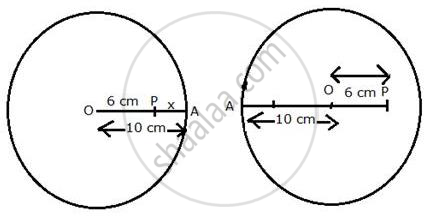Share

# O is the Centre of a Circle of Radius 10 Cm. P is Any Point in the Circle Such that Op = 6 Cm. a is the Point Travelling Along the Circumference. X is the Distance from a to P. What Are the Least and - Mathematics

Course

#### Question

O is the centre of a circle of radius 10 cm. P is any point in the circle such that OP = 6 cm. A is the point travelling along the circumference. x is the distance from A to P. what are the least and the greatest values of x in cm? what is the position of the points O, P and A at these values?

#### Solution

The least value of x will be when A is on OP produced, i.e. O, P and A are collinear.∴ AP = OA - OP = 10 - 6 = 4 cm.
The maximum value of x will be when A is on PO produced, i.e. A, O and P are collinear.
∴ AP = OA + OP = 10 + 6 = 16 cm.

Is there an error in this question or solution?